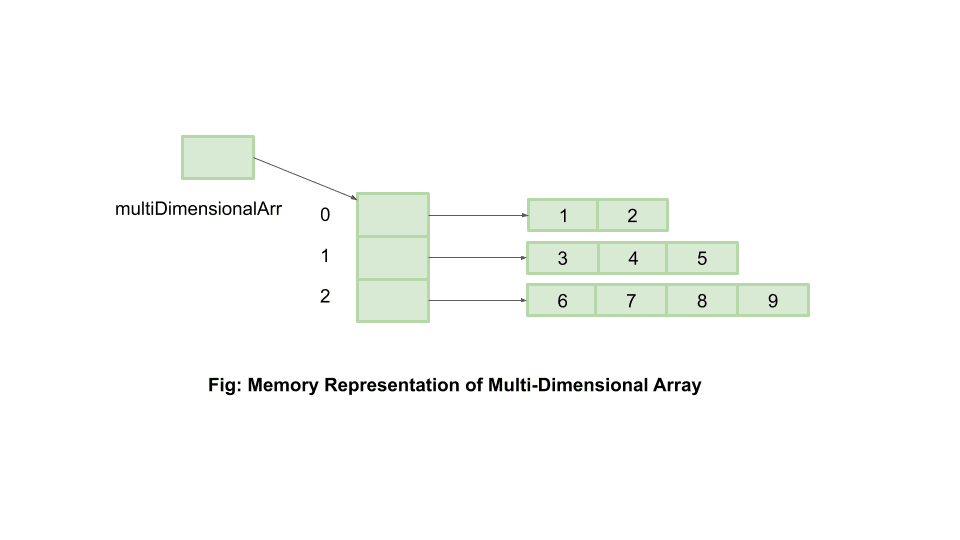### Get started with Spring 5 and Spring Boot 2, through the Learn Spring course:

>> CHECK OUT THE COURSE

## 1. Overview

A multi-dimensional array in Java is an array comprising arrays of varying sizes as its elements. It’s also referred to as “an array of arrays” or “ragged array” or “jagged array”.

In this quick tutorial, we’ll look more in-depth into defining and working with multi-dimensional arrays.

## 2. Creating Multi-Dimensional Array

Let’s start by looking at ways in which we can create a multi-dimensional array:

### 2.1. The Shorthand-Form

An easy way to define a multi-dimensional array would be:

``int[][] multiDimensionalArr = {{1, 2}, {3, 4, 5}, {6, 7, 8, 9}};``

Here, we’ve declared and initialized multiDimensionalArr in a single step.

### 2.2. Declaration and Then Initialization

We start by declaring a multi-dimensional array of size three:

``int[][] multiDimensionalArr = new int[];``

Here, we’ve omitted to specify the second dimension since it will vary.

Next, let’s go further by both declaring and initializing the respective elements within multiDimensionalArr:

``````multiDimensionalArr = new int[] {1, 2};
multiDimensionalArr = new int[] {3, 4, 5};
multiDimensionalArr = new int[] {6, 7, 8, 9};``````

We can also simply declare its elements without initializing them:

``````multiDimensionalArr = new int;
multiDimensionalArr = new int;
multiDimensionalArr = new int;``````

These can then later be initialized, for example by using user inputs.

We can also use the java.util.Arrays.fill method to initialize array elements:

``````void initialize2DArray(int[][] multiDimensionalArray) {
for (int[] array : multiDimensionalArray) {
Arrays.fill(array, 7);
}
}
``````

All the elements in the arrays are initialized with the same value.

## 3. Memory Representation

How will the memory representation of our multiDimensionalArr look like?

As we know, an array in Java is nothing but an object, the elements of which could be either primitives or references. So, a two-dimensional array in Java can be thought of as an array of one-dimensional arrays.

Our multiDimensionalArr in memory would look similar to:Clearly, multiDimensionalArr is holding a reference to a single-dimensional array of size 2, multiDimensionalArr holds a reference to another one-dimensional array of size 3 and so on.

This way Java makes it possible for us to define and use multi-dimensional arrays.

## 4. Iterating Over Elements

We can iterate a multi-dimensional array much like any other array in Java.

Let’s try iterating and initializing the multiDimensionalArr elements using user inputs:

``````void initializeElements(int[][] multiDimensionalArr) {
Scanner sc = new Scanner(System.in);
for (int outer = 0; outer < multiDimensionalArr.length; outer++) {
for (int inner = 0; inner < multiDimensionalArr[outer].length; inner++) {
multiDimensionalArr[outer][inner] = sc.nextInt();
}
}
}``````

Here, multiDimensionalArr[outer].length is the length of an array at an index outer in multiDimensionalArr.

It helps us to ensure that we are looking for elements only within a valid range of each sub-array, thereby avoiding an ArrayIndexOutOfBoundException.

## 5. Printing Elements

What if we want to print the elements of our multi-dimensional array?

One obvious way would be to use the iteration logic we’ve already covered. This involves iterating through each item within our multi-dimensional array, which itself is an array, and then iterating over that child array – one element at a time.

Another option we have is to use java.util.Arrays.toString() helper method:

``````void printElements(int[][] multiDimensionalArr) {
for (int index = 0; index < multiDimensionalArr.length; index++) {
System.out.println(Arrays.toString(multiDimensionalArr[index]));
}
}``````

And we end up having clean and simple code. The generated console output would look like:

``[1, 2] [3, 4, 5] [6, 7, 8, 9]``

## 6. Length of Elements

We can find the length of the arrays in a multi-dimensional array by iterating over the main array:

``````int[] findLengthOfElements(int[][] multiDimensionalArray) {
int[] arrayOfLengths = new int[multiDimensionalArray.length];
for (int i = 0; i < multiDimensionalArray.length; i++) {
arrayOfLengths[i] = multiDimensionalArray[i].length;
}
return arrayOfLengths;
}``````

We can also find the length of arrays using Java streams:

``````Integer[] findLengthOfArrays(int[][] multiDimensionalArray) {
return Arrays.stream(multiDimensionalArray)
.map(array -> array.length)
.toArray(Integer[]::new);
}``````

## 7. Copy a 2-D Array

We can copy a 2-D array using the Arrays.copyOf method:

``````int[][] copy2DArray(int[][] arrayOfArrays) {
int[][] copied2DArray = new int[arrayOfArrays.length][];
for (int i = 0; i < arrayOfArrays.length; i++) {
int[] array = arrayOfArrays[i];
copied2DArray[i] = Arrays.copyOf(array, array.length);
}
return copied2DArray;
}``````

We can also achieve this by using Java streams:

``````Integer[][] copy2DArray(Integer[][] arrayOfArrays) {
return Arrays.stream(arrayOfArrays)
.map(array -> Arrays.copyOf(array, array.length))
.toArray(Integer[][]::new);
}``````

## 8. Conclusion

In this article, we looked at what multi-dimensional arrays are, how they look in-memory and the ways in which we can define and use them.

As always, the source code of the examples presented can be found over on GitHub.

### Get started with Spring 5 and Spring Boot 2, through the Learn Spring course:

>> CHECK OUT THE COURSE